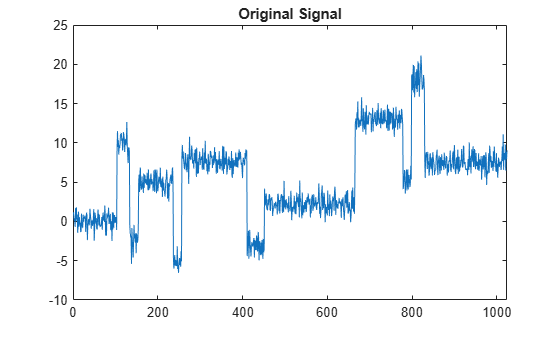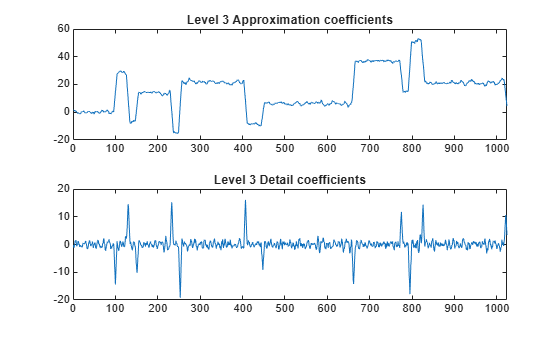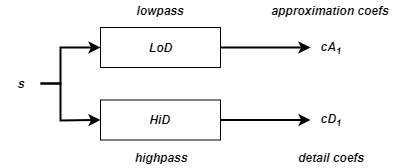# swt

Discrete stationary wavelet transform 1-D

## Syntax

``swc = swt(x,n,wname)``
``swc = swt(x,n,LoD,HiD)``
``[swa,swd] = swt(___)``

## Description

example

````swc = swt(x,n,wname)` returns the stationary wavelet decomposition of the signal `x` at level `n` using the wavelet `wname`. Note`swt` is defined using periodic extension. The length of the approximation and detail coefficients computed at each level equals the length of the signal. ```
````swc = swt(x,n,LoD,HiD)` returns the stationary wavelet decomposition using the specified lowpass and highpass wavelet decomposition filters `LoD` and `HiD`, respectively.```
````[swa,swd] = swt(___)` returns the approximation coefficients `swa` and stationary wavelet coefficients `swd` using either of the previous syntaxes.```

## Examples

collapse all

Perform a multilevel stationary wavelet decomposition of a signal.

Load a one-dimensional signal and acquire its length.

```load noisbloc s = noisbloc; sLen = length(s);```

Perform a stationary wavelet decomposition at level 3 of the signal using `'db1'`. Extract the detail and approximation coefficients at level 3.

```[swa,swd] = swt(s,3,'db1'); swd3 = swd(3,:); swa3 = swa(3,:);```

Plot the output of the decomposition.

```plot(s) xlim([0 sLen]) title('Original Signal')```Plot the level 3 approximation and detail coefficients.

```subplot(2,1,1) plot(swa3) xlim([0 sLen]) title('Level 3 Approximation coefficients') subplot(2,1,2) plot(swd3) xlim([0 sLen]) title('Level 3 Detail coefficients')```## Input Arguments

collapse all

Input signal, specified as a real-valued vector.

Data Types: `double`

Level of decomposition, specified as a positive integer. 2n must divide the length of `x`. Use `wmaxlev` to determine the maximum level of decomposition.

Data Types: `double`

Analyzing wavelet, specified as a character vector or string scalar. `swt` supports only Type 1 (orthogonal) or Type 2 (biorthogonal) wavelets. See `wfilters` for a list of orthogonal and biorthogonal wavelets.

Wavelet decomposition filters, specified as a pair of even-length real-valued vectors. `LoD` is the lowpass decomposition filter, and `HiD` is the highpass decomposition filter. The lengths of `LoD` and `HiD` must be equal. See `wfilters` for additional information.

## Output Arguments

collapse all

Stationary wavelet decomposition, returned as a real-valued matrix. The coefficients are stored row-wise:

• For 1 ≤ i`n`, the ith row of `swc` contains the detail coefficients of level i.

• `swc(n+1,:)` contains the approximation coefficients of level `n`.

Data Types: `double`

Approximation coefficients, returned as a real-valued matrix. For 1 ≤ i`n`, the ith row of `swa` contains the approximation coefficients of level i.

Data Types: `double`

Detail coefficients, returned as a real-valued matrix. For 1 ≤ i`n`, the ith row of `swd` contains the detail coefficients of level i.

Data Types: `double`

## Algorithms

Given a signal s of length N, the first step of the stationary wavelet transform (SWT) produces, starting from s, two sets of coefficients: approximation coefficients cA1 and detail coefficients cD1. These vectors are obtained by convolving s with the lowpass filter `LoD` for approximation, and with the highpass filter `HiD` for detail.

More precisely, the first step iswheredenotes convolution with the filter X.

Note

cA1 and cD1 are of length `N` instead of `N/2` as in the DWT case.

The next step splits the approximation coefficients cA1 in two parts using the same scheme, but with modified filters obtained by upsampling the filters used for the previous step and replacing s by cA1. Then, the SWT produces cA2 and cD2. More generally,where

• F0 = LoD

• G0 = HiD

•— Upsample (insert zeros between elements)

 Nason, G. P., and B. W. Silverman. “The Stationary Wavelet Transform and Some Statistical Applications.” In Wavelets and Statistics, edited by Anestis Antoniadis and Georges Oppenheim, 103:281–99. New York, NY: Springer New York, 1995. https://doi.org/10.1007/978-1-4612-2544-7_17.

 Coifman, R. R., and D. L. Donoho. “Translation-Invariant De-Noising.” In Wavelets and Statistics, edited by Anestis Antoniadis and Georges Oppenheim, 103:125–50. New York, NY: Springer New York, 1995. https://doi.org/10.1007/978-1-4612-2544-7_9.

 Pesquet, J.-C., H. Krim, and H. Carfantan. “Time-Invariant Orthonormal Wavelet Representations.” IEEE Transactions on Signal Processing 44, no. 8 (August 1996): 1964–70. https://doi.org/10.1109/78.533717.